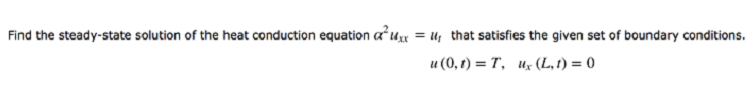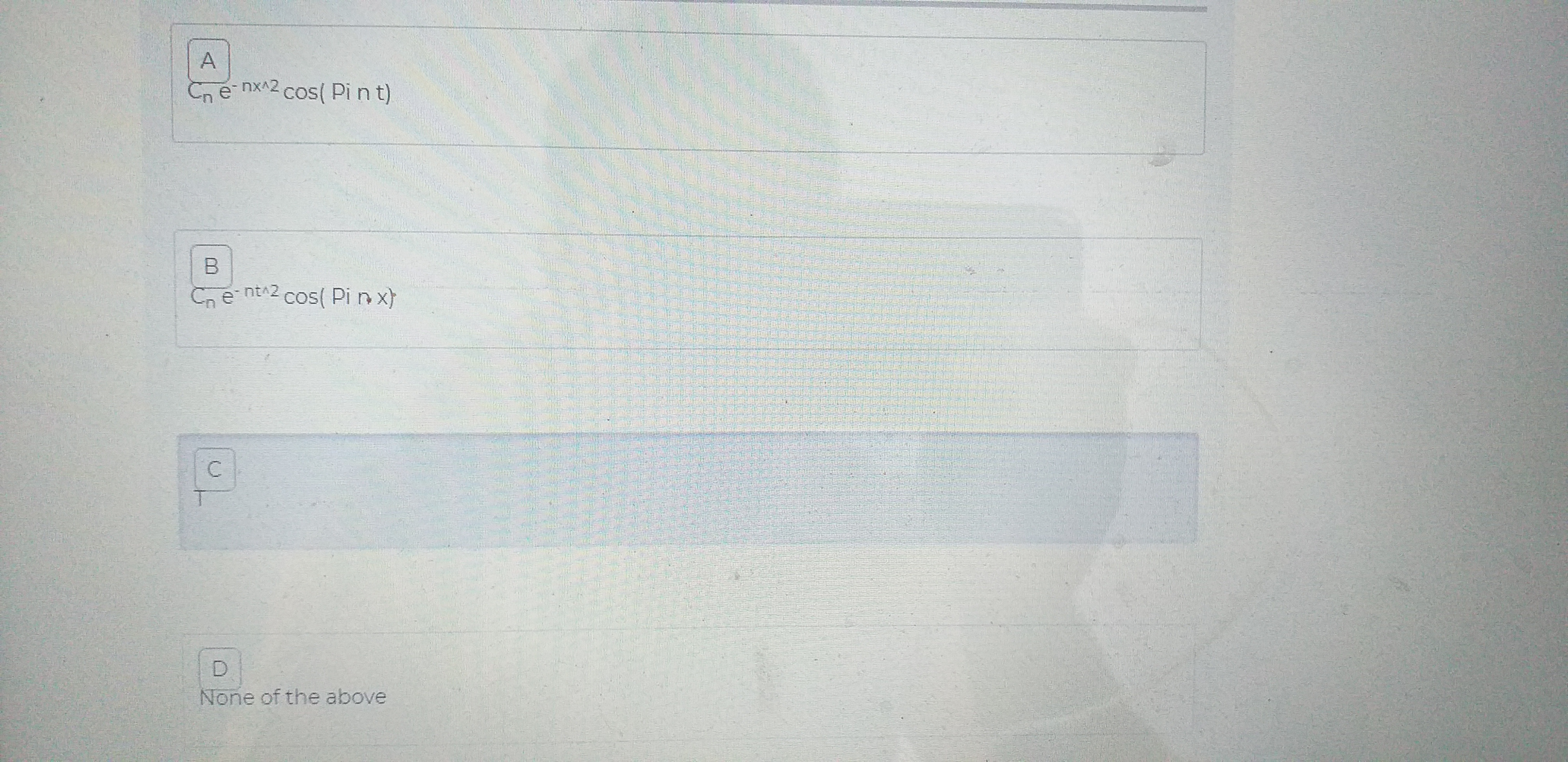# Find the steady-state solution of the heat conduction equation aux = u, that satisfies the given set of boundary conditions.u (L, 1) = 0u(0,)= T, ACn e nx2 cos(Pi n t)BCe nt 2 cos( Pi n xTDNone of the above

Question
6 views

choose the correct optionhelp_outlineImage TranscriptioncloseFind the steady-state solution of the heat conduction equation aux = u, that satisfies the given set of boundary conditions. u (L, 1) = 0 u(0,)= T, fullscreenhelp_outlineImage TranscriptioncloseA Cn e nx2 cos(Pi n t) B Ce nt 2 cos( Pi n x T D None of the above fullscreen
check_circle

Step 1

Given heat conduction equation and the boundary conditions as:

Step 2

By separation method, consider

Step 3

Then equation (1)...

### Want to see the full answer?

See Solution

#### Want to see this answer and more?

Solutions are written by subject experts who are available 24/7. Questions are typically answered within 1 hour.*

See Solution
*Response times may vary by subject and question.
Tagged in